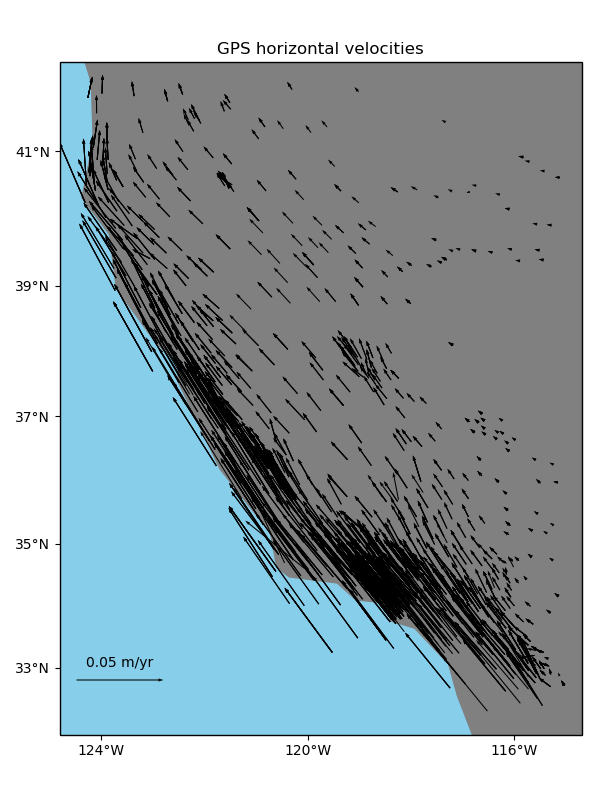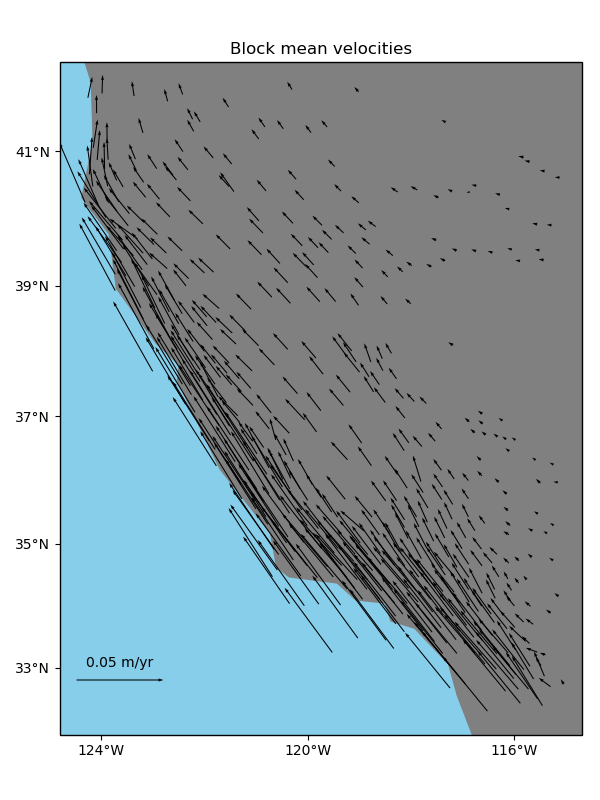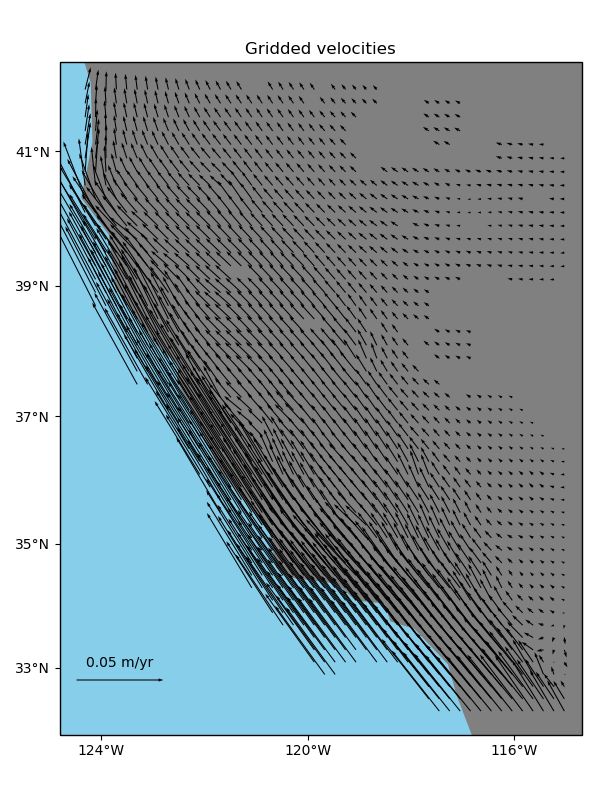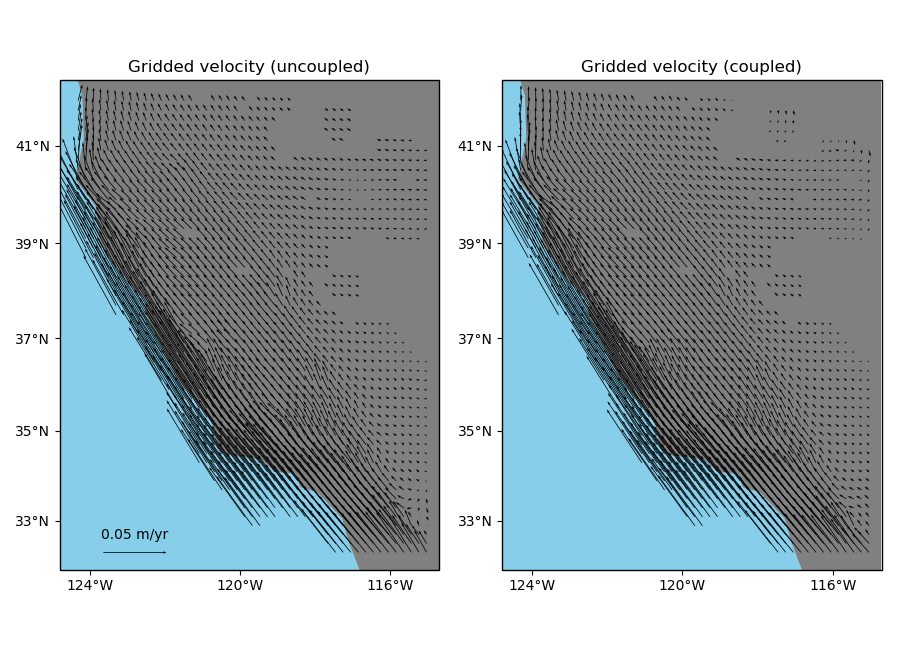# Vector Data¶

Some datasets have multiple vector components measured for each location, like the East and West components of wind speed or GPS velocities. For example, let’s look at our sample GPS velocity data from the U.S. West coast.

import matplotlib.pyplot as plt
import cartopy.crs as ccrs
import pyproj
import verde as vd

data = vd.datasets.fetch_california_gps()

# We'll need to project the geographic coordinates to work with our Cartesian
# classes/functions
projection = pyproj.Proj(proj="merc", lat_ts=data.latitude.mean())
proj_coords = projection(data.longitude.values, data.latitude.values)
# This will be our desired grid spacing in degrees
spacing = 12 / 60

plt.figure(figsize=(6, 8))
ax = plt.axes(projection=ccrs.Mercator())
crs = ccrs.PlateCarree()
tmp = ax.quiver(
data.longitude.values,
data.latitude.values,
data.velocity_east.values,
data.velocity_north.values,
scale=0.3,
transform=crs,
width=0.002,
)
ax.quiverkey(tmp, 0.2, 0.15, 0.05, label="0.05 m/yr", coordinates="figure")
ax.set_title("GPS horizontal velocities")
vd.datasets.setup_california_gps_map(ax)
plt.tight_layout()
plt.show()Verde classes and functions are equipped to deal with vector data natively or through the use of the verde.Vector class. Function and classes that can take vector data as input will accept tuples as the data and weights arguments. Each element of the tuple must be an array with the data values for a component of the vector data. As with coordinates, the order of components must be (east_component, north_component, up_component).

## Blocked reductions¶

Operations with verde.BlockReduce and verde.BlockMean can handle multi-component data automatically. The reduction operation is applied to each data component separately. The blocked data and weights will be returned in tuples as well following the same ordering as the inputs. This will work for an arbitrary number of components.

# Use a blocked mean with uncertainty type weights
reducer = vd.BlockMean(spacing=spacing * 111e3, uncertainty=True)
block_coords, block_data, block_weights = reducer.filter(
coordinates=proj_coords,
data=(data.velocity_east, data.velocity_north),
weights=(1 / data.std_east ** 2, 1 / data.std_north ** 2),
)
print(len(block_data), len(block_weights))


Out:

2 2


We can convert the blocked coordinates back to longitude and latitude to plot with Cartopy.

block_lon, block_lat = projection(*block_coords, inverse=True)

plt.figure(figsize=(6, 8))
ax = plt.axes(projection=ccrs.Mercator())
crs = ccrs.PlateCarree()
tmp = ax.quiver(
block_lon,
block_lat,
block_data,
block_data,
scale=0.3,
transform=crs,
width=0.002,
)
ax.quiverkey(tmp, 0.2, 0.15, 0.05, label="0.05 m/yr", coordinates="figure")
ax.set_title("Block mean velocities")
vd.datasets.setup_california_gps_map(ax)
plt.tight_layout()
plt.show()## Gridding¶

You can use verde.Vector to create multi-component gridders out of verde.Spline the same way as we did for trends. In this case, each component is treated separately.

We can start by splitting the data into training and testing sets (see Model Selection). Notice that verde.train_test_split work for multicomponent data automatically.

train, test = vd.train_test_split(
coordinates=proj_coords,
data=(data.velocity_east, data.velocity_north),
weights=(1 / data.std_east ** 2, 1 / data.std_north ** 2),
random_state=1,
)


Now we can make a 2-component spline. Since verde.Vector implements fit, predict, and filter, we can use it in a verde.Chain to build a pipeline.

We need to use a bit of damping so that the weights can be taken into account. Splines without damping provide a perfect fit to the data and ignore the weights as a consequence.

chain = vd.Chain(
[
("mean", vd.BlockMean(spacing=spacing * 111e3, uncertainty=True)),
("trend", vd.Vector([vd.Trend(1), vd.Trend(1)])),
("spline", vd.Vector([vd.Spline(damping=1e-10), vd.Spline(damping=1e-10)])),
]
)
print(chain)


Out:

Chain(steps=[('mean', BlockMean(adjust='spacing', center_coordinates=False, region=None,
spacing=22200.0, uncertainty=True)), ('trend', Vector(components=[Trend(degree=1), Trend(degree=1)])), ('spline', Vector(components=[Spline(damping=1e-10, engine='auto', force_coords=None, mindist=1e-05), Spline(damping=1e-10, engine='auto', force_coords=None, mindist=1e-05)]))])


Warning

Never generate the component gridders with [vd.Spline()]*2. This will result in each component being a represented by the same Spline object, causing problems when trying to fit it to different components.

Fitting the spline and gridding is exactly the same as what we’ve done before.

chain.fit(*train)

# Score on the test data
print(chain.score(*test))

grid = chain.grid(
region=region,
spacing=spacing,
projection=projection,
dims=["latitude", "longitude"],
)
print(grid)


Out:

0.9926769042771435
<xarray.Dataset>
Dimensions:          (latitude: 49, longitude: 47)
Coordinates:
* longitude        (longitude) float64 235.7 235.9 236.1 ... 244.6 244.8 245.0
* latitude         (latitude) float64 32.29 32.49 32.69 ... 41.5 41.7 41.9
Data variables:
east_component   (latitude, longitude) float64 -0.04659 ... -0.0006166
north_component  (latitude, longitude) float64 0.08792 ... -0.0006041
Attributes:


Mask out the points too far from data and plot the gridded vectors.

grid = vd.distance_mask(
(data.longitude, data.latitude),
maxdist=spacing * 2 * 111e3,
grid=grid,
projection=projection,
)

plt.figure(figsize=(6, 8))
ax = plt.axes(projection=ccrs.Mercator())
tmp = ax.quiver(
grid.longitude.values,
grid.latitude.values,
grid.east_component.values,
grid.north_component.values,
scale=0.3,
transform=crs,
width=0.002,
)
ax.quiverkey(tmp, 0.2, 0.15, 0.05, label="0.05 m/yr", coordinates="figure")
ax.set_title("Gridded velocities")
vd.datasets.setup_california_gps_map(ax)
plt.tight_layout()
plt.show()Another way of gridding 2-component vector data is using verde.VectorSpline2D. This gridder uses linear elasticity theory to couple the two vector components. The degree of coupling can be controlled through the poisson parameter which sets the Poisson’s ratio of the elastic medium.

chain_coupled = vd.Chain(
[
("mean", vd.BlockMean(spacing=spacing * 111e3, uncertainty=True)),
("trend", vd.Vector([vd.Trend(1), vd.Trend(1)])),
("spline", vd.VectorSpline2D(poisson=0.5, damping=1e-10)),
]
)
chain_coupled.fit(*train)
print(chain_coupled.score(*test))


Out:

0.9906070698504343


VectorSpline2D generally gives better results when gridding GPS velocities, particularly for higher density grids and areas with sharp changes in velocity [SandwellWessel2016]. Here, we won’t see a big difference because of the low-density grid that we’re making.

grid_coupled = chain_coupled.grid(
region=region,
spacing=spacing,
projection=projection,
dims=["latitude", "longitude"],
)
(data.longitude, data.latitude),
maxdist=spacing * 2 * 111e3,
grid=grid_coupled,
projection=projection,
)

fig, axes = plt.subplots(
1, 2, figsize=(9, 6.5), subplot_kw=dict(projection=ccrs.Mercator())
)
crs = ccrs.PlateCarree()
titles = ["Gridded velocity (uncoupled)", "Gridded velocity (coupled)"]
grids = [grid, grid_coupled]
for ax, grd, title in zip(axes, grids, titles):
ax.set_title(title)
tmp = ax.quiver(
grd.longitude.values,
grd.latitude.values,
grd.east_component.values,
grd.north_component.values,
scale=0.3,
transform=crs,
width=0.002,
)
vd.datasets.setup_california_gps_map(ax)
ax.quiverkey(tmp, 0.15, 0.15, 0.05, label="0.05 m/yr", coordinates="figure")
plt.tight_layout()
plt.show()Total running time of the script: ( 0 minutes 5.344 seconds)

Gallery generated by Sphinx-Gallery